#### You may also like### Consecutive Numbers

An investigation involving adding and subtracting sets of consecutive numbers. Lots to find out, lots to explore.### Have You Got It?

Can you explain the strategy for winning this game with any target?### Counting Factors

Is there an efficient way to work out how many factors a large number has?

# Sum of 1s

##### Age 11 to 14 ShortChallenge Level

Writing it out in columns
In the units column, there are 2016 1s: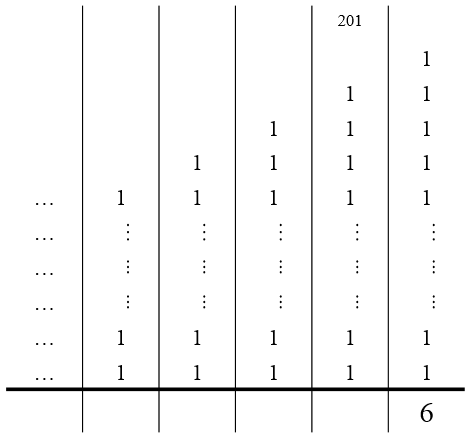In the tens column, there are 2015 1s and 201 carried from before
2015 + 201 = 2216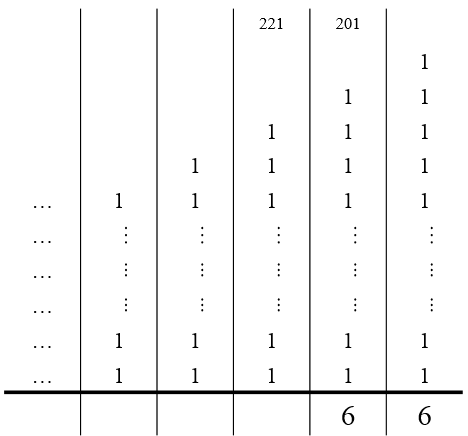In the hundreds column, there are 2014 1s
2014 + 221 = 2235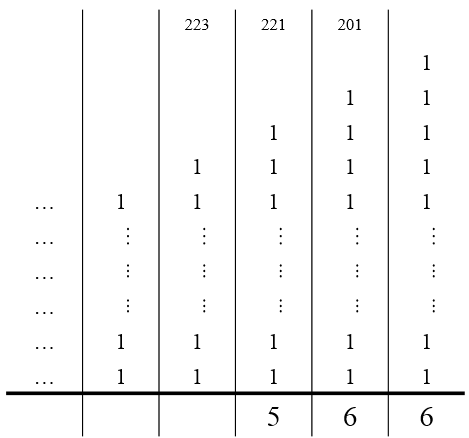Continue: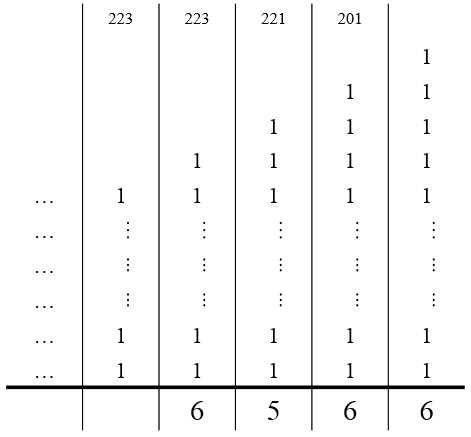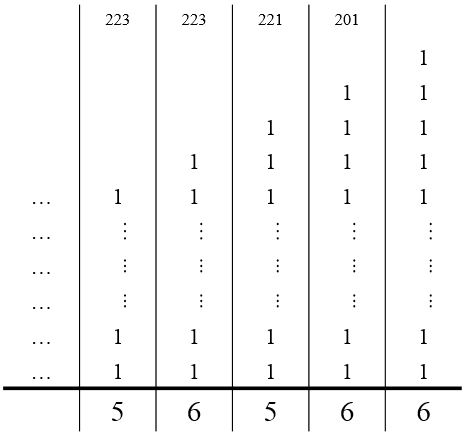Using place value
The units (ones) digit of every number in the list is 1, so there are 2016 units (ones) in the sum.

The first number doesn't have a tens digit, but the tens digit of every other number is 1, so there are 2015 tens from the tens digits in the sum.

And so on.

This is filled in in the table below, which shows the place value of each digit. At the bottom, adding the digits in each of the last five columns gives the last five digits of the sum, which are 56566.

 TTh Th H T U 2 0 1 6 2 0 1 5 2 0 1 4 2 0 1 3 2 0 1 2 5 6 5 6 6

You can find more short problems, arranged by curriculum topic, in our short problems collection.